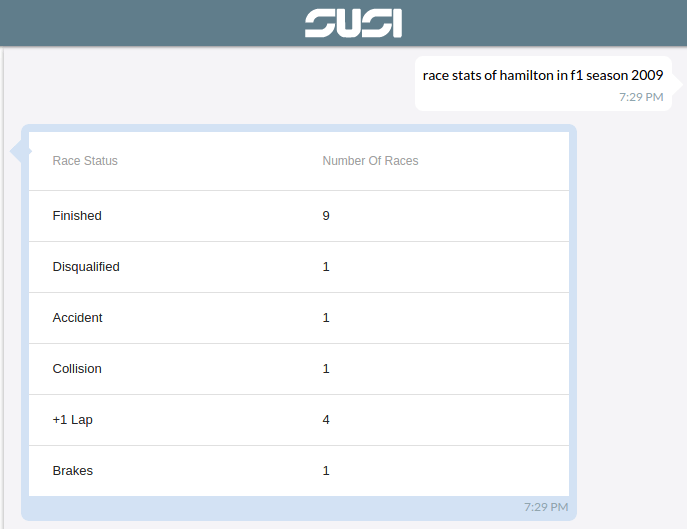# How SUSI AI Tabulates Answers For You

SUSI is an artificial intelligence chat bot that responds to all kinds of user queries. It isn’t any regular chat bot replying in just plain text. It supports various response types which we refer to as ‘actions’. One such action is the “table” type. When the response to a user query contains a list of answers which can be grouped, it is better visualised as a table rather than plain text.

Lets visit SUSI WebChat and try it out. In our example we ask SUSI for the 2009 race statistics of British Formula 1 racing driver Lewis Hamilton.

Query: race stats of hamilton in f1 season 2009

Response: <table> (API response)How does SUSI do that? Let us look at the skill teaching SUSI to give table responses.

```# Returns race stats as a table

race summary of  * in f1 season *|race stats of  * in f1 season *
!console:
{
"url":"http://ergast.com/api/f1/\$2\$/drivers/\$1\$/status.json",
"path":"\$.MRData.StatusTable.Status",
"actions":[{
"type":"table",
"columns":{"status":"Race Status","count":"Number Of Races"}
}]
}
eol
```

Here, we are telling SUSI that the data type is a table through type attribute in actions and also defining column names and under which column each value must be put using their respective keys. Using this information SUSI generates a response accordingly with the table schema and data points.

How do we know when to render a table?

We know it through the type attribute in the actions from the API response.

```"actions": [{
"type": "table",
"columns": {
"status": "Race Status",
"count": "Number Of Races"
},
"count": -1
}]
}],
```

We can see that the type is table so we now know that we have to render a table.

But what is the table schema? What do we fill it with?

There is a columns key under actions and from the value of the columns key we get a object whose key value pairs give us column names and what data to put under each column.

Here, we have two columns – Race Status and Number Of Races

And the data to put under each column is found in answers.data with same keys as those for each column name i.e ‘status’ and ‘count’.

Sample data object from the API response:

```{
"statusId": "2",
"count": "1",
"status": "Disqualified"
}
```

The value under ‘status’ key is ‘Disqualified’ and the column name for ‘status’ key is ‘Race Status’, so Disqualified is entered under Race Status column in the table. Similarly 1  is entered under Number Of Races column. We thus have a row of our table. We populate the table for each object in the data array using the same procedure.

```let coloumns = data.answers.actions[index].columns;
```

We also have a ’count’ attribute in the API response . This is used to denote how many rows to populate in the table. If count = -1 , then it means infinite or to display all the results.

```function drawTable(coloumns,tableData,count){

let parseKeys;
let showColName = true;

if(coloumns.constructor === Array){
parseKeys = coloumns;
showColName = false;
}
else{
parseKeys = Object.keys(coloumns);
}

});

let rowCount = tableData.length;

if(count > -1){
rowCount = Math.min(count,tableData.length);
}

let rows = [];

for (var j=0; j < rowCount; j++) {

let eachrow = tableData[j];

let rowcols = parseKeys.map((key,i) =>{
return(
<TableRowColumn key={i}>
{eachrow[key]}
</TableRowColumn>
);
});

rows.push(
<TableRow key={j}>{rowcols}</TableRow>
);

}

const table =
<MuiThemeProvider>
<Table selectable={false}>
<TableBody displayRowCheckbox={false}>{rows}</TableBody>
</Table>
</MuiThemeProvider>

return table;

}
```

Here we first determine how many rows to populate using the count attribute and then parse the columns to get the column names and keys. We then loop through the data and populate each row.

This is how SUSI responds with tabulated data!

You can create your own table skill and SUSI will give the tabulated response you need. Check out this tutorial to know more about SUSI and the various other action types it supports.

Resources